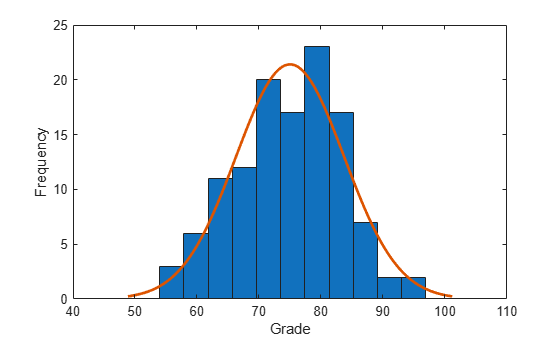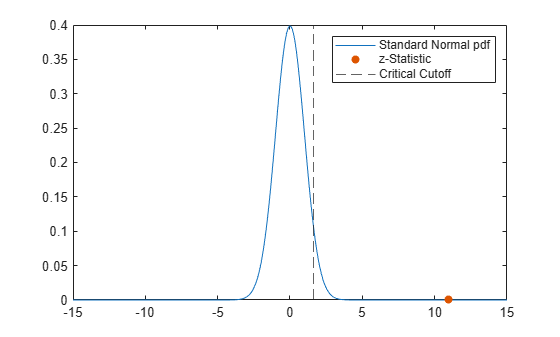# ztest

## Syntax

``h = ztest(x,m,sigma)``
``h= ztest(x,m,sigma,Name,Value)``
``````[h,p] = ztest(___)``````
``````[h,p,ci,zval] = ztest(___)``````

## Description

example

````h = ztest(x,m,sigma)` returns a test decision for the null hypothesis that the data in the vector `x` comes from a normal distribution with mean `m` and a standard deviation `sigma`, using the z-test. The alternative hypothesis is that the mean is not `m`. The result `h` is `1` if the test rejects the null hypothesis at the 5% significance level, and `0` otherwise.```

example

````h= ztest(x,m,sigma,Name,Value)` returns a test decision for the z-test with additional options specified by one or more name-value pair arguments. For example, you can change the significance level or conduct a one-sided test.```

example

``````[h,p] = ztest(___)``` also returns the p-value of the test, using any of the input arguments from previous syntaxes.```

example

``````[h,p,ci,zval] = ztest(___)``` also returns the confidence interval of the population mean, `ci`, and the value of the test statistic, `zval`.```

## Examples

collapse all

Load the sample data. Create a vector containing the first column of the students' exam grades data.

```load examgrades x = grades(:,1);```

Test the null hypothesis that the data comes from a normal distribution with mean `m = 75` and standard deviation `sigma = 10`.

`[h,p,ci,zval] = ztest(x,75,10)`
```h = 0 ```
```p = 0.9927 ```
```ci = 2×1 73.2191 76.7975 ```
```zval = 0.0091 ```

The returned value of `h = 0` indicates that `ztest` does not reject the null hypothesis at the default 5% significance level.

Load the sample data. Create a vector containing the first column of the students' exam grades data.

```load examgrades x = grades(:,1);```

Plot a histogram of the exam grades data and fit a normal density function.

```histfit(x) xlabel("Grade") ylabel("Frequency")```Test the null hypothesis that the data comes from a normal distribution with mean `m = 65` and standard deviation `sigma = 10`, against the alternative hypothesis that the mean is greater than 65.

`[h,~,~,zval] = ztest(x,65,10,"Tail","right")`
```h = 1 ```
```zval = 10.9636 ```

The returned value of `h = 1` indicates that `ztest` rejects the null hypothesis at the default 5% significance level, in favor of the alternate hypothesis that the population mean is greater than 65.

Plot the standard normal distribution, the returned z-statistic, and the critical z-value. Calculate the critical z-value for the default confidence level of 95% by using `norminv`.

```k = linspace(-15,15,300); y = normpdf(k); zvalpdf = normpdf(zval); zcrit = norminv(0.95); plot(k,y); hold on scatter(zval,zvalpdf,"filled") xline(zcrit,"--") legend(["Standard Normal pdf","z-Statistic", ... "Critical Cutoff"])```The orange dot represents the z-statistic and is located to the right of the dashed black line that represents the critical z-value.

## Input Arguments

collapse all

Sample data, specified as a vector, matrix, or multidimensional array.

• If `x` is specified as a vector, `ztest` returns a single value for each output argument.

• If `x` is specified as a matrix, `ztest` performs a separate z-test along each column of `x` and returns a vector of results.

• If `x` is specified as a multidimensional array, `ztest` works along the first nonsingleton dimension of `x`.

In all cases, `ztest` treats `NaN` values as missing data and ignores them.

Data Types: `single` | `double`

Hypothesized mean, specified as a scalar value.

Data Types: `single` | `double`

Population standard deviation, specified as a scalar value.

Data Types: `single` | `double`

### Name-Value Arguments

Specify optional pairs of arguments as `Name1=Value1,...,NameN=ValueN`, where `Name` is the argument name and `Value` is the corresponding value. Name-value arguments must appear after other arguments, but the order of the pairs does not matter.

Before R2021a, use commas to separate each name and value, and enclose `Name` in quotes.

Example: `'Tail','right','Alpha',0.01` specifies a right-tailed hypothesis test at the 1% significance level.

Significance level of the hypothesis test, specified as the comma-separated pair consisting of `'Alpha'` and a scalar value in the range (0,1).

Example: `'Alpha',0.01`

Data Types: `single` | `double`

Dimension of the input matrix along which to test the means, specified as the comma-separated pair consisting of `'Dim'` and a positive integer value. For example, specifying `'Dim',1` tests the column means, while `'Dim',2` tests the row means.

Example: `'Dim',2`

Data Types: `single` | `double`

Type of alternative hypothesis to evaluate, specified as the comma-separated pair consisting of `'Tail'` and one of:

• `'both'` — Test against the alternative hypothesis that the population mean is not `m`.

• `'right'` — Test against the alternative hypothesis that the population mean is greater than `m`.

• `'left'` — Test against the alternative hypothesis that the population mean is less than `m`.

`ztest` tests the null hypothesis that the population mean is `m` against the specified alternative hypothesis.

Example: `'Tail','right'`

## Output Arguments

collapse all

Hypothesis test result, returned as `1` or `0`.

• If `h` `= 1`, this indicates the rejection of the null hypothesis at the `Alpha` significance level.

• If `h` `= 0`, this indicates a failure to reject the null hypothesis at the `Alpha` significance level.

p-value of the test, returned as a scalar value in the range [0,1]. `p` is the probability of observing a test statistic as extreme as, or more extreme than, the observed value under the null hypothesis. Small values of `p` cast doubt on the validity of the null hypothesis.

Confidence interval for the true population mean, returned as a two-element vector containing the lower and upper boundaries of the 100 × (1 – `Alpha`)% confidence interval.

Test statistic, returned as a nonnegative scalar value.

collapse all

### z-Test

The z-test is a parametric hypothesis test used to determine whether a sample data set comes from a population with a particular mean. The test assumes that the sample data comes from a population with a normal distribution and a known standard deviation.

The test statistic is

`$z=\frac{\overline{x}-\mu }{\sigma /\sqrt{n}},$`

where $\overline{x}$ is the sample mean, `μ` is the population mean, σ is the population standard deviation, and n is the sample size. Under the null hypothesis, the test statistic has a standard normal distribution.

### Multidimensional Array

A multidimensional array has more than two dimensions. For example, if `x` is a 1-by-3-by-4 array, then `x` is a three-dimensional array.

### First Nonsingleton Dimension

The first nonsingleton dimension is the first dimension of an array whose size is not equal to 1. For example, if `x` is a 1-by-2-by-3-by-4 array, then the second dimension is the first nonsingleton dimension of `x`.

## Tips

• Use `sampsizepwr` to calculate:

• The sample size that corresponds to specified power and parameter values;

• The power achieved for a particular sample size, given the true parameter value;

• The parameter value detectable with the specified sample size and power.

## Version History

Introduced before R2006a